#### 期刊菜单

Conversion and Comparison between Isobaric, Isothermal and Isosteric Adsorption of Meager Coal
DOI: 10.12677/JAPC.2022.112006, PDF, HTML, XML, 下载: 72  浏览: 258

Abstract: Based on five groups of adsorption data of lean coal in Xiangshan mine, Shaanxi Province, the parameters A, B, ∆ and β are obtained by regression calculation through temperature-pressure-adsorption equation. It is verified by the relative average error and the comparison between the measured points and the fitting surface. The adsorption pressures required for lean coal at different adsorption temperatures are calculated when the adsorption amount is 5.0 cm3/g. Press lnp~1/T to draw a straight line. The negative slope of the line indicates that the adsorption is an exothermic process. According to Clausius Clapeyron definite integral equation, the adsorption process is an exothermic process. In order to maintain the same adsorption capacity at high temperature, the gas pressure must be increased, that is, if T2 > T1, P2 > P1. It is proved by isobaric, isothermal and isokinetic adsorption lines.

1. 引言

2. 贫煤等温吸附实验数据Table 1. Measured conditions, Langmuir volume and Langmuir pressure parameters of coal samples

3. 温度–压力–吸附方程和克劳修斯–克拉佩龙方程

$\text{V}=\frac{1}{\sqrt{\text{MT}}}\left[\text{A}+{\text{BT}}^{1.5}{\text{P}}^{\text{β}}\mathrm{exp}\left(\frac{\Delta }{\text{T}}\right)\right]$ (1)

A：吸附介质几何形体常数；

B：吸附流量系数，无量纲；

M：分子量，甲烷为16；

P：测试时的压力，兆帕；

T：测试时的热力学温度，K；

V：吸附量，cm3/g；

B：压力影响的参数，无量纲；

∆：温度影响的参数，K。

TPAE的四个参数A、B、∆和β是四个待定参数。在方程1中，温度与压力为自变量，吸附量V为因变量。先确定压力值，可以得到吸附量与温度在恒压条件下的关系，运用方程1计算不同温度下的吸附值。固定温度值，可以计算在不同压力下的吸附值，得到吸附量与压力之间的关系。

$\text{lnP}=\frac{1}{\text{β}}\left[\mathrm{ln}\left(\text{V}\sqrt{\text{MT}}-\text{A}\right)-\mathrm{ln}\left({\text{BT}}^{1.5}\right)\right]-\frac{1}{\text{β}}\frac{\Delta }{\text{T}}$ (2)

$\mathrm{ln}\text{p}=\frac{{\Delta }_{\text{g}}^{\text{l}}{\text{H}}_{\text{m}}}{\text{R}}\frac{1}{\text{T}}+\text{C}$ (3)

${\Delta }_{\text{g}}^{\text{l}}{\text{H}}_{\text{m}}$ ：摩尔吸附焓；

M：摩尔；

G：始态；

L：终态；

R：气体常数，J/(mol∙K)；

C积分常数项。

$-{\Delta }_{\text{g}}^{\text{l}}{\text{H}}_{\text{m}}=\frac{{\text{RT}}_{2}{\text{T}}_{1}}{{\text{T}}_{2}-{\text{T}}_{1}}\mathrm{ln}\frac{{\text{P}}_{2}}{{\text{P}}_{1}}$ (4)

4. 结果与讨论

4.1. TPAE的参数回归Table 2. TPAE parameters regressed according to Langmuir volume and Langmuir pressure parameters in Table 1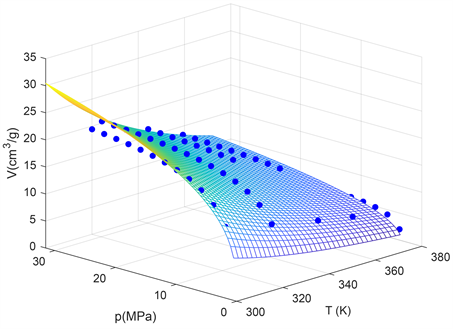Figure 1. Measured points of lean coal and TPAE regression surface

4.2. 等量吸附焓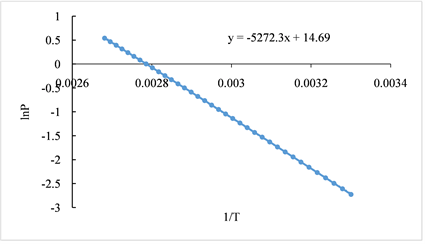Figure 2. lnp~1/T diagram of equal 5 cm3/g adsorption capacity of lean coal

4.3. 吸附等温线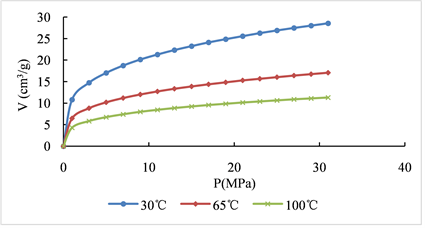Figure 3. Adsorption isotherms at three different temperatures

4.4. 吸附等压线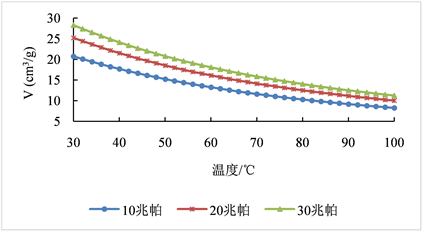Figure 4. Adsorption isobars under three different pressures

4.5. 吸附等量线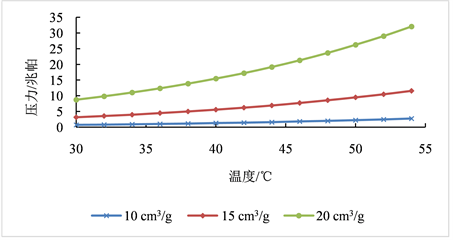5. 结语

  赵丽娟, 秦勇, Geoff Wang, 等. 高温高压条件下深部煤层气吸附行为[J]. 高校地质学报, 2013, 19(4): 648-654.  马东明, 张遂安, 蔺亚兵. 煤的等温吸附解吸实验及其精确拟合[J]. 煤炭学报, 2011, 36(3): 476-480.  傅雪海, 秦勇, 权彪, 等. 中煤级煤吸附甲烷的物理模拟与数值模拟研究[J]. 地质学报, 2008, 82(10): 1368-1371.  秦勇, 申建, 王宝文, 等. 深部煤层气成藏效应及其耦合关系[J]. 石油学报, 2012, 33(1): 48-54.  申建, 秦勇, 傅雪海, 等. 深部煤层气成藏条件特殊性及其临界深度探讨[J]. 天然气地球科学, 2014, 25(9): 1470-1476.  李东, 郝静远. 无机膜气体分离的温度-压力-渗透率方程及其在吸附上的应用[J]. 膜科学与技术, 2018, 38(4): 127-131.  李东, 张学梅, 马青华, 郝静远. 基于煤岩分析及温度-压力-吸附量方程预测煤层气量的软件V1.0 [CP/CD]. 著作权登记号: 2019SR0722572.  李东, 张学梅, 郝静远, 马青华. 基于吸附验证的煤层气含量的可行性研究[J]. 煤炭科学技术, 2018, 46(9): 27-31.  张学梅, 李东. 变温变压下煤层气吸附量的预测[J]. 化工进展, 2018, 37(s1): 63-66.  张学梅, 马青华, 郝静远, 等. 努尔森扩散对崔家沟煤温度压力吸附所作贡献的数值分析[J]. 煤质技术, 2019, 34(3): 20-22.  李东, 张学梅, 郝静远, 等. 温度-压力-吸附方程在计算煤岩等量吸附焓的应用[J]. 化工进展, 2019, 38(s1): 104-109.  张学梅, 李东, 马青华, 等. 单位等量解吸焓对煤与瓦斯突出综合作用假说的补充[J]. 煤矿安全, 2021, 52(7): 155-161.  李东, 郝静远, 张学梅, 等. 陕西焦坪崔家沟煤岩吸附热力学特征分析[J]. 低温与特气, 2018, 36(1): 16-19.  李东, 郝静远, 张学梅, 马青华. 温度-压力-吸附方程回归样本集的建立与计算-以陕西焦坪崔家沟煤为例[J]. 非常规油气, 2018, 5(2): 46-49.